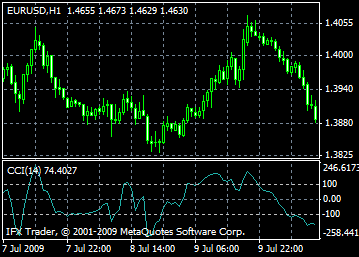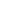# Commodity Channel Index - CCI

Commodity Channel Index ( CCI) is a technical indicator, which allows traders and analysts to detect overbought and oversold levels. It means that after some calculations, one can measure deviation of instrument price from the average price. If the analyzed instrument price touches the highest value, there is a strong uptrend on the market. In case the index is below the lowest value, it is a sell signal.

Initially CCI was developed to reveal volatility on raw material markets, but today Commodity Channel Index is applied to any financial instrument.

Nowadays analysts and traders use Commodity Channel Index to determine two main signals.

1. Divergences. Divergences appear when price indicator hits the highest score, but the indicator is unable to rise above the previous highs. Consequently, if the index increases and the price falls, the price is bound to start an upward movement. But if the index lowers and the price increases, the price decline is to start.

2. Overbought and oversold levels. If the index value is beyond ±100, it indicates that the financial instrument is overbought or oversold. In case the value is above +100, corrective decline is possible, but in case the value is below -100, there is a probability of a corrective rise.## Calculation

1. To find a typical price, you need to add the HIGH, the LOW, and the CLOSE prices of each bar and then divide the result by 3.

TP = (HIGH + LOW +CLOSE)/3

2. To calculate the n-period Simple Moving Average of typical prices.

SMA(TP, N) = SUM[TP, N]/N

3. To subtract the received SMA(TP, N) from Typical Prices.

D = TP - SMA(TP, N)

4. To calculate the n-period Simple Moving Average of absolute D values.

SMA(D, N) = SUM[D, N]/N

5. To multiply the received SMA(D, N) by 0,015.

M = SMA(D, N) * 0,015

6. To divide M by D

CCI = M/D

where

SMA - Simple Moving Average;

N - number of periods, used for calculation.

All MT5 content